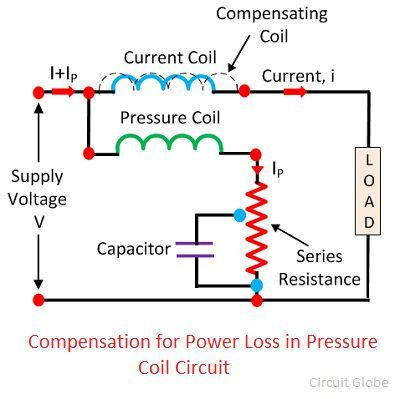# Low Power Factor Wattmeter

Definition: The instrument that measures the low value of power factor accurately is known as the Low Power Factor Wattmeter (LPFW). The low power factor meter is used for measuring the power of the highly inductive circuit. It is also used in the resistive circuit which has a power factor range from 0.5 to 1.

## What is the need of LPFW?

The ordinary Wattmeter used for measuring the low power factor gives the inaccurate result. This happens because of two reasons.

1. In low power factor meter, the magnitude of deflecting torque on moving coil is small even after the full excitation of the pressure and current coil.
2. The error occurs in the reading because of the pressure coil inductance.

Some additional features are added on the ordinary Wattmeter so that the meter can measure the power of the low power factor circuit.

## Modifications in Ordinary Wattmeter

### Pressure Coil Current

The pressure coil is designed for having a low value of resistance so that the high value of current passes through it. This current produces the deflecting torque on the moving coil.

### Compensation for Pressure Coil

There are two substitute methods of connecting the Wattmeter in the circuit. The figure below shows these two methods.In figure (a) the pressure coil is parallel connected to the supply voltage. And the current coil is connected in series with the supply voltage. The magnitude of the voltage across the pressure coil is equal to the supplied voltage. The total power measured by the pressure coil is equal to the sum of the power loss in the load and the power loss in the current coil.In figure (b) the pressure coil is not connected in parallel with the load. Thereby, the magnitude of the pressure coil voltage is not equal to the supplied voltage. The output power obtains from the circuit is equal to the sum of the load power loss and the power loss of the pressure coil.

In low power circuit, the value of current is high, and that of the power is low. The high-value current causes the error in the Wattmeter reading. For reducing the error, the compensation coil is used in the circuit. The compensating coil compensates the error in the circuit which induces because of low power factor.The compensating coil and the pressure coil are in series with each other. The coil is coordinate with the current coil. The pressure coil current Ip flows through it. The field induces in the compensating coil is because of the current Ip. The field of the compensating coil and the current coil opposes each other. Thus the error occurs in the pressure coil is neutralized.

### Compensation For Inductance of Pressure Coil

The small amount of inductances is present in the pressure coil of the Wattmeter. This inductance causes the error in the reading. The error occurs in the pressure coil is given by the expression

VISinφtanβ

The φ is the angle between the pressure and the current coil. For a small value power factor φ is large. The large value of φ causes a large error in the reading. The compensating coil is used in the circuit for compensating the inductance error occurs in the Wattmeter. Along with the compensating coil, the capacitor is used in the circuit. The capacitor is placed in parallel with the pressure coil resistance.The capacitor eliminates the error. The compensation caused by the capacitor is slightly affected by the variation in frequencies. Above 10kHz the compensation is accurately held in the Wattmeter.

### Small Control Torque

The control torque of the low power factor Wattmeter is kept small so that the full-scale deflection is obtained even for the small value of the power factor.

### 1 thought on “Low Power Factor Wattmeter”

1. In low power circuit, the value of current is high, and that of the power is low. I can’t understand this , please explain